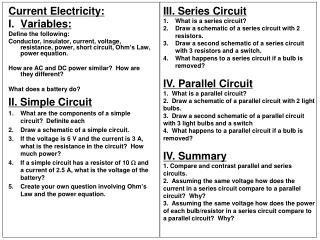DownloadDownload PresentationCurrent Electricity: Variables: Define the following:

# Current Electricity: Variables: Define the following:

Télécharger la présentation## Current Electricity: Variables: Define the following:

- - - - - - - - - - - - - - - - - - - - - - - - - - - E N D - - - - - - - - - - - - - - - - - - - - - - - - - - -
##### Presentation Transcript

1. III. Series Circuit • What is a series circuit? • Draw a schematic of a series circuit with 2 resistors. • Draw a second schematic of a series circuit with 3 resistors and a switch. • What happens to a series circuit if a bulb is removed? • IV. Parallel Circuit • What is a parallel circuit? • Draw a schematic of a parallel circuit with 2 light bulbs. • Draw a second schematic of a parallel circuit with 3 light bulbs and a switch • What happens to a parallel circuit if a bulb is removed? • IV. Summary • Compare and contrast parallel and series circuits. • Assuming the same voltage how does the current in a series circuit compare to a parallel circuit? Why? • Assuming the same voltage how does the power of each bulb/resistor in a series circuit compare to a parallel circuit? Why? Current Electricity: • Variables: Define the following: Conductor, insulator, current, voltage, resistance, power, short circuit, Ohm’s Law, power equation. How are AC and DC power similar? How are they different? What does a battery do? II. Simple Circuit • What are the components of a simple circuit? Definite each • Draw a schematic of a simple circuit. • If the voltage is 6 V and the current is 3 A, what is the resistance in the circuit? How much power? • If a simple circuit has a resistor of 10 W and a current of 2.5 A, what is the voltage of the battery? • Create your own question involving Ohm’s Law and the power equation.